Overview

This is a demonstration of regularized mediation analysis methods based on penalized structural equation modeling. The original version (version 1.x) on CRAN was for structural equations with multiple mediators between a single exposure variable and a single outcome variable. The penalized model implemented in the regmed functions is based on sparse group lasso in which the pair of parameters alpha and beta are considered a group; alpha is the effect of exposure on a mediator and beta is the effect of that mediator on the outcome. Version 2.x and later include now include computationally efficient models that allow for multiple exposures, multiple mediators, and multiple outcomes. The penalized model for this multivariate situation, implemented in functions denoted mvregmed, uses a lasso (L1) type of penalty for all parameters: alpha that link exposures with mediators; beta that link mediators with outcomes; delta that link exposures directly with outcomes.

The default way of analyzing the data is to center and scale all x, mediator, and y variables, so each column of the x, mediator, and y variables has mean 0 and variance 1. This default approach is recommended, because it places all variables on the same scale. If adjustment for extraneous covariates is needed, regression of any of the variables on covaraites should be performed prior to use of regmed or mvregmed, and the residuals from these regression models can be used as input variables to the methods of regmed or mvregmed. When standardized varibles are used, the penalized structural equation models are modeling the correlations of variables.

Simulated dataset

The simulated data set (named “medsim”) contains 100 subjects with 10 exposure “x” variables, 200 mediator “med” variables, and 2 outcome “y” variables.

regmed: Mediation model for 1 exposure, multiple mediators, and 1 outcome variable

This section focuses on the original version of regmed that handles a single exposure and a single outcome. The user user-level functions for single outcome/exposure are:

• regmed.prefilter() pre-filters mediators if there are more than the number of subjects
• regmed.grid() penalized model for grid of lambdas
• regmed.grid.bestfit() get best fit model from grid of fits
• regmed.fit() fit penalized model with specified lambda
• plot.regmed.grid() plot results of regmed.grid
• regmed.edges() create edges from a fit of a model for use in plotting and model fit by lavaan
• plot.regmed.edges() plot directed graph based on edges: exposure -> mediator -> outcome
• regmed.lavaan.model() setup model to estimate unpenalized parameters by lavaann sem function
• regmed.lavaan.dat() setup data to input tolavaann sem function
• summary.lavaan() summarize lavaan sem model fit

Pre-filter mediators

If the number of mediators exceeds the sample size, model fitting can become unstable. To reduce the number of mediators to fit, the regmed.prefilter uses sure independence screening (Fan & Lv, 2008) to reduce the number of potential mediator. This is based on ranking marginal correlations and then selecting the highest ranked values such that the number of parameters is less than the sample size. Because mediation depends on the two correlations, $$cor(x,med)$$ and $$cor(med, y)$$ we rank the absolute values of their products, $$|cor(x, med) * cor(med, y)|$$, and choose the highest k ranked values to determine which potential mediators to include in penalized mediation models. If k is not specified, the default value of k is n/2, where n is the sample size, because each mediator results in two parameters alpha and beta.

We choose k=10 mediators to select, to speed calculations solely for demonstration purposes. Also, we select the first exposure variable and the first outcome variable to demonstrate methods for regmed. The function regmed.prefilter returns a list of x, mediator, and y, with each of these variables centered and scaled by default, and accounts for missing data by subsetting to subjects that are not missing any of the variables.

Grid of lambda penalties

To fit a series of models over a grid of penalty “lambda” values, it is necessary to define a vector of lambda values. It is best to arrange this vector from largest to smallest lambda values to assure that the largest lambda (first value in the vector) results in no selected parameters (alpha, beta, delta), and the smallest lambda (last value in the vector) selects multiple parameters, and that a lambda between the largest and smallest results in a minimum Bayesian Information Criterion (BIC). Starting with a large lambda and then decreasing lambda values is best, because this provides a “warm start” for each subsequent fit, with final fitted parameter values for a specific lambda used as initial values for the next lambda value. The demonstration below extracts variables that have been processed by regmed.prefilter.

Fit regmed for grid of lambda penalties

fitting grid lambda [ 1 ] =  0.4
fitting grid lambda [ 2 ] =  0.39
fitting grid lambda [ 3 ] =  0.38
fitting grid lambda [ 4 ] =  0.37
fitting grid lambda [ 5 ] =  0.36
fitting grid lambda [ 6 ] =  0.35
fitting grid lambda [ 7 ] =  0.34
fitting grid lambda [ 8 ] =  0.33
fitting grid lambda [ 9 ] =  0.32
fitting grid lambda [ 10 ] =  0.31
fitting grid lambda [ 11 ] =  0.3
fitting grid lambda [ 12 ] =  0.29
fitting grid lambda [ 13 ] =  0.28
fitting grid lambda [ 14 ] =  0.27
fitting grid lambda [ 15 ] =  0.26
fitting grid lambda [ 16 ] =  0.25
fitting grid lambda [ 17 ] =  0.24
fitting grid lambda [ 18 ] =  0.23
fitting grid lambda [ 19 ] =  0.22
fitting grid lambda [ 20 ] =  0.21
fitting grid lambda [ 21 ] =  0.2
fitting grid lambda [ 22 ] =  0.19
fitting grid lambda [ 23 ] =  0.18
fitting grid lambda [ 24 ] =  0.17
fitting grid lambda [ 25 ] =  0.16
fitting grid lambda [ 26 ] =  0.15
fitting grid lambda [ 27 ] =  0.14
fitting grid lambda [ 28 ] =  0.13
fitting grid lambda [ 29 ] =  0.12
fitting grid lambda [ 30 ] =  0.11
fitting grid lambda [ 31 ] =  0.1
fitting grid lambda [ 32 ] =  0.09
fitting grid lambda [ 33 ] =  0.08
fitting grid lambda [ 34 ] =  0.07
fitting grid lambda [ 35 ] =  0.06
fitting grid lambda [ 36 ] =  0.05
fitting grid lambda [ 37 ] =  0.04
fitting grid lambda [ 38 ] =  0.03
fitting grid lambda [ 39 ] =  0.02
fitting grid lambda [ 40 ] =  0.01

The plot method for regmed.grid gives two figures that represent the size of the coefficients over the grid of lambdas, and the BIC by by the grid of lambdas.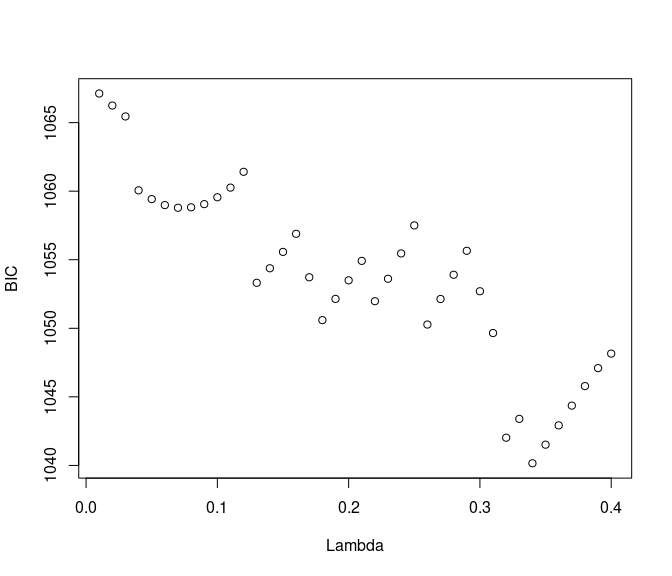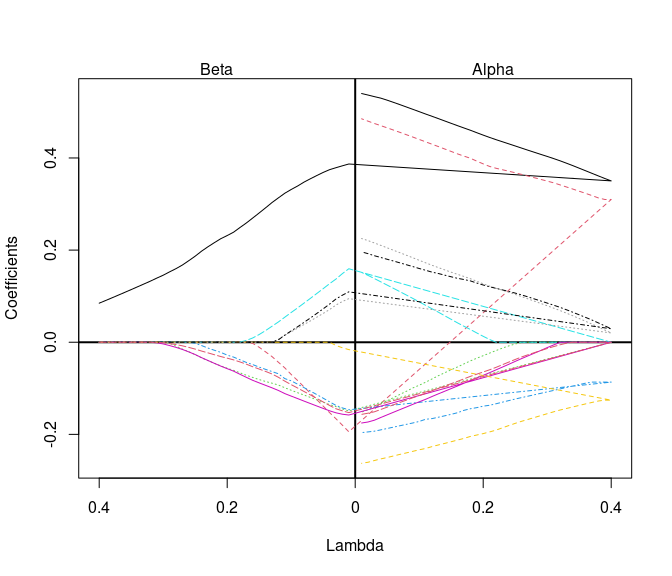Select best model from grid by minimum BIC

Call:
NULL

Coefficients:
alpha      beta alpha*beta
med.1    0.38341929 0.1203989 0.04616325
med.2    0.33255320 0.0000000 0.00000000
med.74   0.00000000 0.0000000 0.00000000
med.88  -0.09504961 0.0000000 0.00000000
med.90   0.00000000 0.0000000 0.00000000
med.99   0.00000000 0.0000000 0.00000000
med.112 -0.14050034 0.0000000 0.00000000
med.129  0.05748298 0.0000000 0.00000000
med.133  0.06508961 0.0000000 0.00000000
med.178  0.00000000 0.0000000 0.00000000

sum of alpha*beta =  0.04616325
delta =  0.5046204
sum of delta + alpha*beta =  0.5507836
var(x) =  1
var(y) =  1.102819

Fit single regmed model with user-specified lambda

If user prefers to specify a lambda penalty and not search through a grid, the function regmed.fit is available.

Call:
regmed.fit(x = x1, mediator = med, y = y1, lambda = 0.3, frac.lasso = 0.8)

Coefficients:
alpha         beta   alpha*beta
med.1    0.40109014  0.126420020 5.070582e-02
med.2    0.35582882  0.000000000 0.000000e+00
med.74   0.00000000  0.000000000 0.000000e+00
med.88  -0.13906482  0.000000000 0.000000e+00
med.90   0.00000000  0.000000000 0.000000e+00
med.99  -0.01045770 -0.001413514 1.478211e-05
med.112 -0.17587944  0.000000000 0.000000e+00
med.129  0.07206755  0.000000000 0.000000e+00
med.133  0.07530145  0.000000000 0.000000e+00
med.178 -0.01914775  0.000000000 0.000000e+00

sum of alpha*beta =  0.05072061
delta =  0.5386689
sum of delta + alpha*beta =  0.5893895
var(x) =  1
var(y) =  1.116486

Plot directed graph of fit model

The demonstration below shows how to determined edges, where an edge is defined by vertex-1 pointing to vertex-2, and the vertices are the variables selected in a model. For example, if alpha and beta are both estimated to be non-zero, then x -> med and med -> y. The function regmed.edges has an option to choose how vertices and edges are selected. By type=“mediators”, vertices and edges are selected only of the product $$alpha * beta$$ is non-zero, because this is required for a mediator to be truely mediating. By specifying type = “any”, all vertices and edges that represent non-zero parameters are selected. For example, if alpha is non-zero, and beta is zero, the edge x -> med is selected, but the edge med -> y is not selected. To determine if terms are zero, a threshold variable eps is used (see help for regmed.edges).

For fit.best, the summary above shows that only med.1 is selected as a mediator, because $$|alpha * beta| > eps$$. In this case, choosing type=“mediators” selects only med.1 to be included in edges.

In contrast, choosing type=“mediators” for fit.best results in including med.2 as an edge, even thouth it is not a mediator.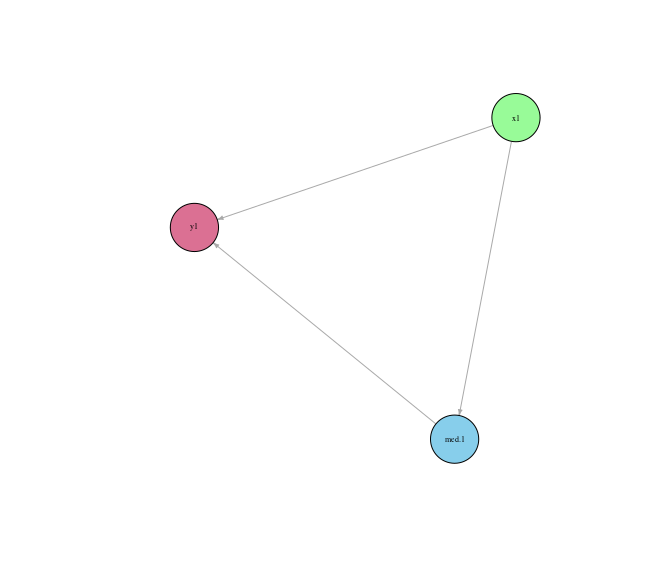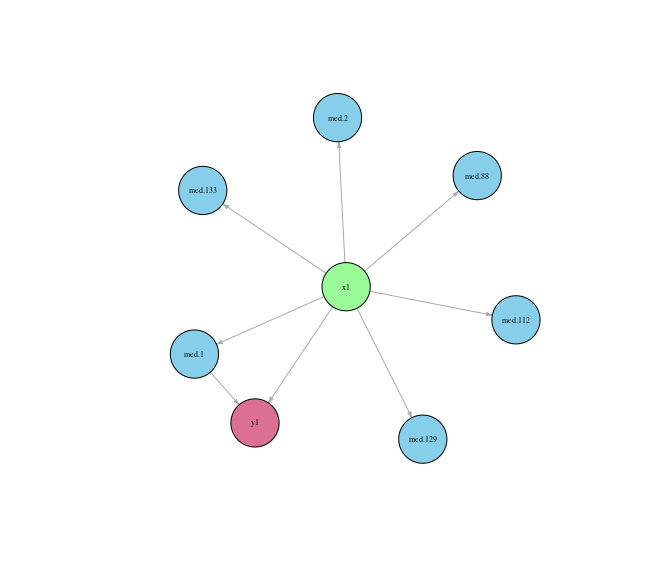Lavaan sem: Estimate parameters of selected model without imposing penalty

Because penalized models can overly shink parameter estimates towards zero, it can be beneficial to refit the selected model without penalties. The model without penalties is a starndard structural equation model, which can be fit with the function sem from the package lavaan. To facilitate this step, the function regmed.lavaan.model uses the edges and fit of a model to create a text string that represents the model syntax for sem. This function abides by our assumption that the residual covariances between x and med, between x and y, and between med and y, are all zero. It also assumes that the covariances of the mediators, a matrix in the fit of a regmed object, are fixed in the sem model fitting.

The demonstration below shows how to setup the models for sem as well as a data set. The function regmed.lavaan.dat assures that x, med, y, are centered and scaled and subset to subjects without any missing data.

The demonstration below shows how to use lavaan sem to fit specified models and view resuts

lhs op   rhs   est    se     z pvalue ci.lower ci.upper
1 med.1  ~    x1 0.551 0.084 6.577  0.000    0.387    0.716
2    y1  ~ med.1 0.293 0.093 3.147  0.002    0.110    0.475
3    y1  ~    x1 0.415 0.093 4.445  0.000    0.232    0.598
4    y1 ~~    y1 0.602 0.085 7.071  0.000    0.435    0.769
lhs op   rhs    est    se      z pvalue ci.lower ci.upper
1   med.1  ~    x1  0.551 0.084  6.577  0.000    0.387    0.716
2   med.2  ~    x1  0.489 0.088  5.576  0.000    0.317    0.661
3  med.88  ~    x1 -0.197 0.099 -2.002  0.045   -0.390   -0.004
4 med.112  ~    x1 -0.264 0.097 -2.723  0.006   -0.454   -0.074
5 med.129  ~    x1  0.232 0.098  2.369  0.018    0.040    0.423
6 med.133  ~    x1  0.200 0.098  2.032  0.042    0.007    0.393
7      y1  ~ med.1  0.293 0.093  3.147  0.002    0.110    0.475
8      y1  ~    x1  0.415 0.093  4.445  0.000    0.232    0.598
9      y1 ~~    y1  0.602 0.085  7.071  0.000    0.435    0.769

mvregmed : Mediation model for multiple exposures, multiple mediators, and multiple outcome variables

New in version 2.0 is the multivariate version mvregmed, mv for multivariate. The user-level functions available for these are listed as follows:

• mvregmed.grid() penalized model for grid of lambdas
• mvregmed.grid.bestfit() get best fit mvregmed model from grid of fits
• mvregmed.fit() fit penalized mvregmed model with specified lambda
• plot.mvregmed.grid() plot results of mvregmed.grid
• plot.mvregmed() plot results of a single mv regmed model fit, from either mvregmed.fit or mvregmed.grid.bestfit
• mvregmed.edges() create edges from a fit of a model for use in plotting and model fit by lavaan
• plot.mvregmed.edges() plot directed graph based on edges: exposure -> mediator -> outcome
• regmed.lavaan.model() setup model to estimate unpenalized parameters by lavaann sem function
• regmed.lavaan.dat() setup data to input to lavaann sem function
• summary.lavaan() summarize lavaan sem model fit, which applies to both regmed.fit and mvregmed.fit runs in the lavaan::sem, because the outputs are the same.

Fit mvregmed for a grid for lambda penalties

With a specified lambda.grid, fit a grid of models over the multiple exposures and mediators. We see in the summary and the plot that a lambda around 0.10 provides the best model.

fitting grid lambda [ 1 ] =  0.4
fitting grid lambda [ 2 ] =  0.39
fitting grid lambda [ 3 ] =  0.38
fitting grid lambda [ 4 ] =  0.37
fitting grid lambda [ 5 ] =  0.36
fitting grid lambda [ 6 ] =  0.35
fitting grid lambda [ 7 ] =  0.34
fitting grid lambda [ 8 ] =  0.33
fitting grid lambda [ 9 ] =  0.32
fitting grid lambda [ 10 ] =  0.31
fitting grid lambda [ 11 ] =  0.3
fitting grid lambda [ 12 ] =  0.29
fitting grid lambda [ 13 ] =  0.28
fitting grid lambda [ 14 ] =  0.27
fitting grid lambda [ 15 ] =  0.26
fitting grid lambda [ 16 ] =  0.25
fitting grid lambda [ 17 ] =  0.24
fitting grid lambda [ 18 ] =  0.23
fitting grid lambda [ 19 ] =  0.22
fitting grid lambda [ 20 ] =  0.21
fitting grid lambda [ 21 ] =  0.2
fitting grid lambda [ 22 ] =  0.19
fitting grid lambda [ 23 ] =  0.18
fitting grid lambda [ 24 ] =  0.17
fitting grid lambda [ 25 ] =  0.16
fitting grid lambda [ 26 ] =  0.15
fitting grid lambda [ 27 ] =  0.14
fitting grid lambda [ 28 ] =  0.13
fitting grid lambda [ 29 ] =  0.12
fitting grid lambda [ 30 ] =  0.11
fitting grid lambda [ 31 ] =  0.1
fitting grid lambda [ 32 ] =  0.09
fitting grid lambda [ 33 ] =  0.08
fitting grid lambda [ 34 ] =  0.07
fitting grid lambda [ 35 ] =  0.06
fitting grid lambda [ 36 ] =  0.05
fitting grid lambda [ 37 ] =  0.04
fitting grid lambda [ 38 ] =  0.03
fitting grid lambda [ 39 ] =  0.02
fitting grid lambda [ 40 ] =  0.01
lambda converge iter  df df.alpha df.beta df.delta df.vary      bic
1    0.40     TRUE    1   3        0       0        0       3 1654.792
2    0.39     TRUE    1   3        0       0        0       3 1654.792
3    0.38     TRUE    1   3        0       0        0       3 1654.792
4    0.37     TRUE    1   3        0       0        0       3 1654.792
5    0.36     TRUE    1   3        0       0        0       3 1654.792
6    0.35     TRUE    1   3        0       0        0       3 1654.792
7    0.34     TRUE    2   4        0       0        1       3 1658.532
8    0.33     TRUE   16   4        0       0        1       3 1654.905
9    0.32     TRUE   20   4        0       0        1       3 1650.588
10   0.31     TRUE   17   4        0       0        1       3 1646.938
11   0.30     TRUE   20   6        1       1        1       3 1648.360
12   0.29     TRUE   18   6        1       1        1       3 1640.782
13   0.28     TRUE   15   7        2       1        1       3 1637.233
14   0.27     TRUE   13   7        2       1        1       3 1628.011
15   0.26     TRUE   11   7        2       1        1       3 1619.421
16   0.25     TRUE   10   8        2       2        1       3 1615.833
17   0.24     TRUE   13   8        2       2        1       3 1606.546
18   0.23     TRUE   13   8        2       2        1       3 1597.676
19   0.22     TRUE   12   8        2       2        1       3 1589.353
20   0.21     TRUE   11   8        2       2        1       3 1581.557
21   0.20     TRUE   10   8        2       2        1       3 1574.278
22   0.19     TRUE    9   8        2       2        1       3 1567.483
23   0.18     TRUE    9   9        2       3        1       3 1565.120
24   0.17     TRUE   10  10        2       4        1       3 1562.287
25   0.16     TRUE   10  12        3       5        1       3 1562.820
26   0.15     TRUE   10  14        4       5        2       3 1562.427
27   0.14     TRUE    9  16        5       6        2       3 1562.163
28   0.13     TRUE    9  19        8       6        2       3 1564.848
29   0.12     TRUE    8  20        9       6        2       3 1557.575
30   0.11     TRUE    8  23       10       6        4       3 1559.310
31   0.10     TRUE    9  24       10       7        4       3 1551.370
32   0.09     TRUE    7  27       11       8        5       3 1553.866
33   0.08     TRUE    8  30       13       9        5       3 1556.457
34   0.07     TRUE   13  38       16      11        8       3 1578.555
35   0.06     TRUE   14  40       16      13        8       3 1572.406
36   0.05     TRUE   16  48       21      14       10       3 1593.056
37   0.04     TRUE   14  59       30      14       12       3 1622.029
38   0.03     TRUE   13  74       43      14       14       3 1661.687
39   0.02     TRUE   13  92       56      16       17       3 1712.467
40   0.01     TRUE   17 120       82      18       17       3 1809.103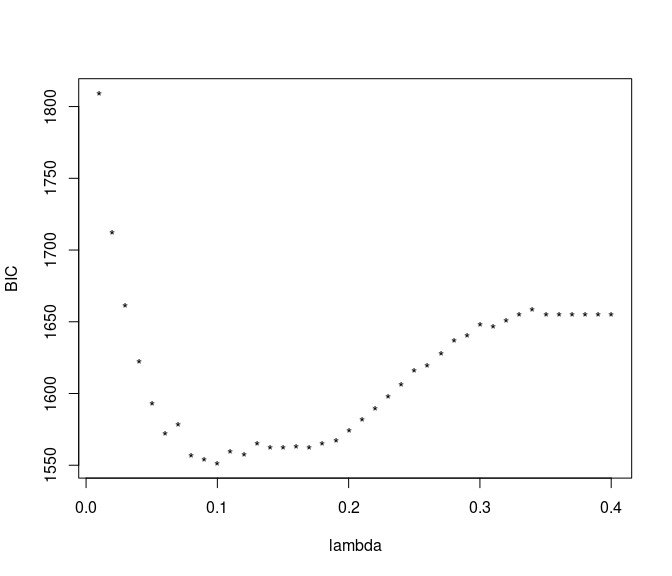Select best model from grid based on minimum BIC

Choose best fit model from grid based on min BIC.

Instead of examining all contents of a model, use summary to view main non-zero parameters from alpha, beta, and delta.

=== alpha parameter estimates ===
mediator   x       alpha
1     med.1 x.1  0.35439717
2     med.1 x.5  0.05232707
3     med.2 x.1  0.30707983
4    med.88 x.1 -0.08543541
5   med.112 x.1 -0.09557552
6   med.112 x.2 -0.03397326
7   med.129 x.1  0.04703450
8   med.129 x.2  0.03646951
9   med.133 x.1  0.02059390
10  med.133 x.5  0.06844645
=== beta parameter estimates ===
y mediator        beta
1 y.1    med.1  0.27605124
2 y.1   med.74 -0.07297674
3 y.1   med.88 -0.04104814
4 y.1   med.90  0.01331543
5 y.1   med.99 -0.09497897
6 y.1  med.178 -0.05289736
7 y.2    med.2  0.26795394
=== delta parameter estimates ===
y    x       delta
1 y.1  x.1  0.27186184
2 y.1  x.5  0.01699286
3 y.1  x.8  0.05065293
4 y.1 x.10 -0.04389265

Fit single mvregmed model with user-specified lambda

Below shows how to fit a single model, and what happens with summary when all alpha, beta, and delta are 0.

Warning in summary.mvregmed(mvfit): all alpha, beta, delta are zero
NULL

Plot directed graph of fit model

After edges are created, we use the pakage igraph to create plots. Because igraph generates graphs based on random seed, it is imporatnt to set a seed to assure the plot can be recreated. We provide the simple function plot.mvregmed.edges to create a basic plot.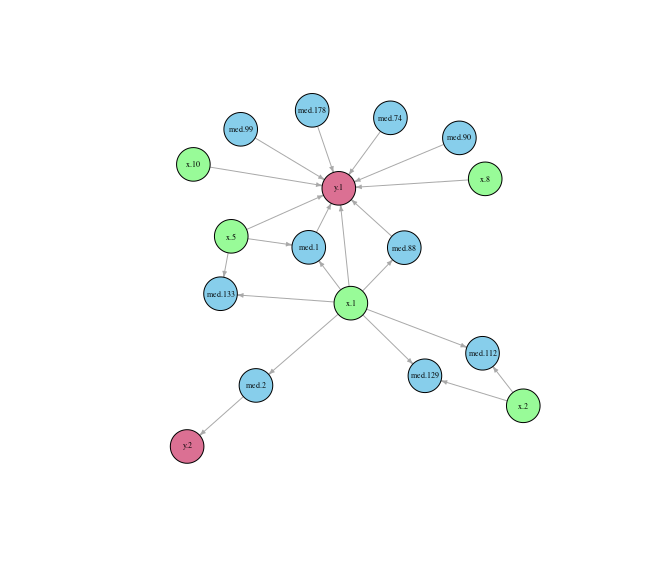To have more control of igraph attributes, we demonstrate below how to use our function mvregmed.graph.attributes to set up initial graph attributes, and then set a variety of igraph attributes in a standard plot function. See igraph for more plot attributes.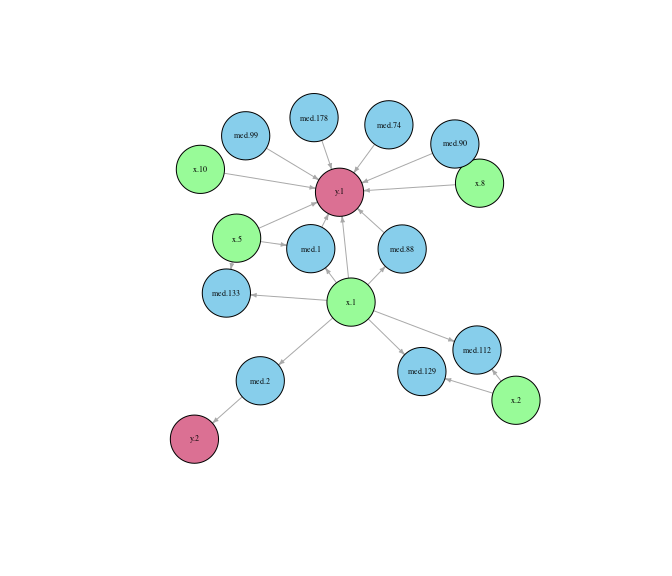Lavaan sem: Estimate parameters of seleted model without imposing penalty

Like methods for regmed, we provide parallel methods for mvregmed that can be used to setup and fit models with lavaan sem. The 4 key steps are:

• determine edges with mvregmed.edges
• create the model with mvregmed.lavaan.model
• setup the data with mvregmed.lavaan.dat
• fit model with sem
lhs op     rhs    est    se      z pvalue ci.lower ci.upper
1    med.1  ~     x.1  0.476 0.086  5.552  0.000    0.308    0.643
2    med.1  ~     x.5  0.174 0.085  2.041  0.041    0.007    0.341
3    med.2  ~     x.1  0.473 0.075  6.283  0.000    0.325    0.620
4   med.88  ~     x.1 -0.196 0.090 -2.172  0.030   -0.373   -0.019
5  med.112  ~     x.1 -0.164 0.106 -1.545  0.122   -0.373    0.044
6  med.112  ~     x.2 -0.141 0.105 -1.338  0.181   -0.348    0.066
7  med.129  ~     x.1  0.175 0.108  1.629  0.103   -0.036    0.386
8  med.129  ~     x.2  0.160 0.106  1.506  0.132   -0.048    0.368
9  med.133  ~     x.1  0.157 0.097  1.619  0.105   -0.033    0.346
10 med.133  ~     x.5  0.219 0.094  2.320  0.020    0.034    0.404
11     y.1  ~     x.1  0.280 0.102  2.745  0.006    0.080    0.480
12     y.1  ~     x.5  0.099 0.082  1.203  0.229   -0.062    0.261
13     y.1  ~     x.8  0.122 0.091  1.342  0.180   -0.056    0.299
14     y.1  ~    x.10 -0.248 0.079 -3.129  0.002   -0.403   -0.093
15     y.1  ~   med.1  0.317 0.089  3.557  0.000    0.143    0.492
16     y.1  ~  med.74 -0.140 0.076 -1.849  0.064   -0.288    0.008
17     y.1  ~  med.88 -0.114 0.075 -1.527  0.127   -0.261    0.032
18     y.1  ~  med.90  0.124 0.072  1.725  0.085   -0.017    0.265
19     y.1  ~  med.99 -0.158 0.072 -2.181  0.029   -0.299   -0.016
20     y.1  ~ med.178 -0.120 0.077 -1.569  0.117   -0.271    0.030
21     y.2  ~   med.2  0.399 0.093  4.267  0.000    0.216    0.582
22     y.1 ~~     y.1  0.468 0.066  7.071  0.000    0.338    0.598
23     y.2 ~~     y.2  0.820 0.116  7.071  0.000    0.593    1.047
24     y.1 ~~     y.2 -0.100 0.063 -1.594  0.111   -0.223    0.023

Interpretation of lavaan output

The parameters alpha, beta, and delta are determined by regression models indicated by the tilde ($$\sim$$) operator, while variance and covariance parameters are indicated by the double tilder ($$\sim\sim$$) operator. Note that the covariance matrices for x and mediator are assumed fixed based on the values returned from regemd and mvregmed, so only variances and covariances for outcome y are provided. Also note that the z values and p-values from lavaan are marginal effects, not from a joint test of model parameters.